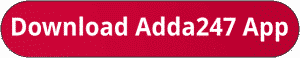Latest Banking jobs   »   bank exam 2023

# Quantitative Aptitude Quiz For Bank Mains Exam 2023-27th October

Directions (1-4): The following questions are accompanied by two statements I and II. You have to determine which statements(s) is/are sufficient/necessary to answer the questions.
Q1. Find the sum of present age of X & Z?
I. Four years ago, average age of X, Y and Z was 16 years while age of X, Y and Z is in arithmetic progression.
II. Average of present age of X and Z is same as present age of Y while Y is four years younger than Z.
(a) Statement I alone is sufficient to answer the question but statement II alone is not sufficient to answer the question.
(b) Statement II alone is sufficient to answer the question but statement I alone is not sufficient to answer the question.
(c) Both the statements taken together are necessary to answer the questions, but neither of the statements alone is sufficient to answer the question.
(d) Either statement I or statement II by itself is sufficient to answer the question.
(e) Statements I and II taken together are not sufficient to answer the question.

Q2. Is Rahul meet Prabhat at half of the distance on a circular track (48km) if they both started to move at same time in same direction?
I. Speed of Prabhat is 24km/hr more than speed of Rahul.
II. Prabhat’s speed is 200% more than that of Rahul
(a) Statement I alone is sufficient to answer the question but statement II alone is not sufficient to answer the question.
(b) Either statement I or statement II by itself is sufficient to answer the question.
(c) Both the statements taken together are necessary to answer the questions, but neither of the statements alone is sufficient to answer the question.
(d) Statement II alone is sufficient to answer the question but statement I alone is not sufficient to answer the question.
(e) Statements I and II taken together are not sufficient to answer the question.

Q3. Find number of yellow balls in a box which contains 3 black balls and 7 red and yellow balls.
I. Probability of selecting two yellow balls from the box is 2/15
II. Probability of selecting one red and black ball is 1/5.
(a) Statement I alone is sufficient to answer the question but statement II alone is not sufficient to answer the question.
(b) Statement II alone is sufficient to answer the question but statement I alone is not sufficient to answer the question.
(c) Both the statements taken together are necessary to answer the questions, but neither of the statements alone is sufficient to answer the question.
(d) Either statement I or statement II by itself is sufficient to answer the question.
(e) Statements I and II taken together are not sufficient to answer the question.

Q4. Find number of pens sold by retailer if he earns total 37.5% profit on selling some pens and some pencils.
I. Ratio between pen sold to pencil sold is 2 : 3 while ratio between cost price of pen to pencil is 3 : 2.
II. On selling a pen and a pencil, he earns 25% and 50% profit respectively.
(a) Both the statements taken together are necessary to answer the questions, but neither of the statements alone is sufficient to answer the question.
(b) Statements I and II taken together are not sufficient to answer the question.
(c) Statement II alone is sufficient to answer the question but statement I alone is not sufficient to answer the question.
(d) Statement I alone is sufficient to answer the question but statement II alone is not sufficient to answer the question.
(e) Either statement I or statement II by itself is sufficient to answer the question.

Q5. Find the share of Satish in profit after one year among Satish, veer and Yogesh
A. Investment of Veer, Satish and Yogesh is in the ratio 6: 8: 9 and share of Yogesh in profit is Rs.900
B. Satish and Veer invested Rs.8000 and Rs.6000 for 9 months and one year respectively. Difference b/w profit earned by Satish and Veer is Zero.
C. Satish, Veer and Yogesh invested for 9 months, 1 year and 8 months respectively and share of Veer in profit is 900.
(a) Either A and B or B and C
(b) Either A and B or A and C
(c) Any two of them
(d) Either B alone or A and C together
(e) Either A and C or B and C

Solutions.                                                                                       .

## FAQs

### When is the IBPS Clerk Mains exam scheduled for?

The IBPS Clerk Mains exam is scheduled for 7 October 2023.

#### Congratulations!Union Budget 2023-24: Free PDF